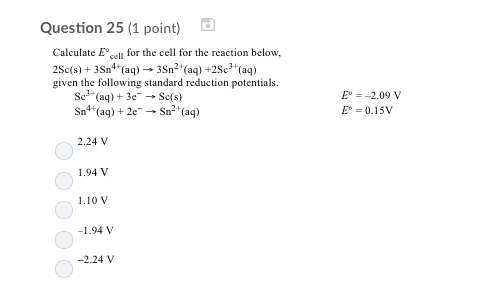# Question & Answer: Calculate E degree_cell for the cell for the reaction below, 2Sc(s) + 3sn^4+(aq) rightarrow…..Calculate E degree_cell for the cell for the reaction below, 2Sc(s) + 3sn^4+(aq) rightarrow 3Sn^2+(aq) + 2Sc^3+(aq) given the following standard reduction potentials. Sc^3+(aq) + 3e^- rightarrow Sc(s) E degree = -2.09 V Sn^4+(aq) + 2e^- rightarrow Sn^2+(aq) E degree = 0.15V 2.24V 1.94 V 1.10 V -1.94 V -2.24 V

Considering the given overall reaction

Don't use plagiarized sources. Get Your Custom Essay on
Question & Answer: Calculate E degree_cell for the cell for the reaction below, 2Sc(s) + 3sn^4+(aq) rightarrow…..
GET AN ESSAY WRITTEN FOR YOU FROM AS LOW AS \$13/PAGE

We can write the standard half reactions accordingly

The given first half reaction is reversed and multiply it by 2

Oxidation reaction

2Sc – – – > 2Sc3+ + 6e- E°ox = +2.09 V

Reduction reaction (multiply by 3)

3 Sn4+ + 6e- – – – – – – > 3 Sn2+ E°red = 0.15 V

Overall reaction

2Sc + 3Sn4+ – – – – – – > 3Sn2+ + 2Sc3+

E°cell = E°ox + E°red = 2.09+0.15 = +2.24 V

Option A is the correct answer.